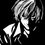# Generalizations!

Whenever I solve a problem, I try if I can proceed on with the generalized statement (or expression) for the problem.

I came up with two interesting generalizations today.

• The straight forward one - The number of ordered n-tuples of integers $\{ x_i \}_{i=1}^n$ such that $\sum_{i=1}^n x_i - \prod_{i=1}^n x_i = n-1$ is equal to $nk-n+1$ provided that $1 \leq x_i \leq k$

 

• This one generalizes the type of problems where you need to find sum of binomial coefficients which are at certain gaps. By gaps I mean, suppose you need to find $\sum_{k=0}^7 {30 \choose 4k}$ as you can find here that binomial coefficients are at certain gaps of $4$. (I hope I am able to explain my point clearly). So here's the generalization - for $m,n \in \mathbb{N}$ such that $m \leq n$ we have

$\sum_{k=0}^{mk \leq n} {n \choose mk} = \dfrac{2^n}{m} \sum_{k=0}^{m-1} \cos^{n} \left(\frac{\pi k}{m}\right) e^{i\frac{nk}{m} \pi}$

  

Exercise -

• Evaluate $\sum_{k=1}^{10} {30 \choose 3k}$

• Prove $\sum_{k=0}^{n-1} (-1)^k \cos^n\left(\frac{\pi k}{n}\right) = \frac{n}{2^{n-1}}$

• Prove both the generalizations.Note by Kishlaya Jaiswal
5 years, 11 months ago

This discussion board is a place to discuss our Daily Challenges and the math and science related to those challenges. Explanations are more than just a solution — they should explain the steps and thinking strategies that you used to obtain the solution. Comments should further the discussion of math and science.

When posting on Brilliant:

• Use the emojis to react to an explanation, whether you're congratulating a job well done , or just really confused .
• Ask specific questions about the challenge or the steps in somebody's explanation. Well-posed questions can add a lot to the discussion, but posting "I don't understand!" doesn't help anyone.
• Try to contribute something new to the discussion, whether it is an extension, generalization or other idea related to the challenge.

MarkdownAppears as
*italics* or _italics_ italics
**bold** or __bold__ bold
- bulleted- list
• bulleted
• list
1. numbered2. list
1. numbered
2. list
Note: you must add a full line of space before and after lists for them to show up correctly
paragraph 1paragraph 2

paragraph 1

paragraph 2

[example link](https://brilliant.org)example link
> This is a quote
This is a quote
    # I indented these lines
# 4 spaces, and now they show
# up as a code block.

print "hello world"
# I indented these lines
# 4 spaces, and now they show
# up as a code block.

print "hello world"
MathAppears as
Remember to wrap math in $$ ... $$ or $ ... $ to ensure proper formatting.
2 \times 3 $2 \times 3$
2^{34} $2^{34}$
a_{i-1} $a_{i-1}$
\frac{2}{3} $\frac{2}{3}$
\sqrt{2} $\sqrt{2}$
\sum_{i=1}^3 $\sum_{i=1}^3$
\sin \theta $\sin \theta$
\boxed{123} $\boxed{123}$

Sort by:

For "sum of binomial coefficients which are at certain gaps", I think that the best approach is using Roots of Unity instead of trying to prove it by induction.

Staff - 5 years, 11 months ago

Yes Sir, indeed that's the method even I used to prove it and henceforth tagged this post with RootsOfUnity filter ;)

- 5 years, 11 months ago

Just say a few words on how do we use roots of unity..

- 4 years, 3 months ago